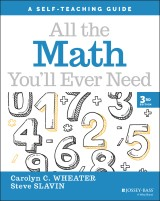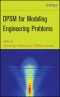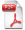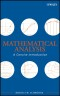# Details## All the Math You'll Ever Need

A Self-Teaching Guide
Wiley Self-Teaching Guides 3. Aufl.
 17,99 € Verlag: Wiley Format: PDF Veröffentl.: 13.04.2022 ISBN/EAN: 9781119719229 Sprache: englisch Anzahl Seiten: 336

DRM-geschütztes eBook, Sie benötigen z.B. Adobe Digital Editions und eine Adobe ID zum Lesen.

## Beschreibungen

#### Titelbeschreibung

<p><b>A comprehensive and hands-on guide to crucial math concepts and terminology</b> <p>In the newly revised third edition of <i>All the Math You’ll Ever Need: A Self-Teaching Guide</i>, veteran math and computer technology teacher Carolyn Wheater and veteran mathematics author Steve Slavin deliver a practical and accessible guide to math you can use every day and apply to a wide variety of life tasks. From calculating monthly mortgage payments to the time you’ll need to pay off a credit card, this book walks you through the steps to understanding basic math concepts. <p>This latest edition is updated to reflect recent changes in interest rates, prices, and wages, and incorporates information on the intelligent and efficient use of calculators and mental math techniques. It also offers: <ul> <li>A brand-new chapter on hands-on statistics to help readers understand common graphs</li> <li>An easy-to-use-format that provides an interactive method with frequent questions, problems, and self-tests</li> <li>Complete explanations of necessary mathematical concepts that explore not just <i>how</i> math works, but also <i>why </i>it works</li></ul><p>Perfect for anyone seeking to make practical use of essential math concepts and strategies in their day-to-day life, <i>All the Math You’ll Ever Need</i> is an invaluable addition to the libraries of students who want a bit of extra help applying math in the real world.

#### Inhaltsverzeichnis

<p>How to Use This Book vii</p> <p>Acknowledgments</p> <p><b>1 Getting Started </b></p> <p><b>2 Essential Arithmetic </b></p> <p>Addition</p> <p>What Is Multiplication?,</p> <p>Subtraction</p> <p>Division</p> <p><b>3 Focus on  Multiplication </b></p> <p>The Multiplication Table,</p> <p>Learning the Multiplication Table,</p> <p>Testing Your Knowledge,</p> <p>About Long Multiplication,</p> <p>Practice Exercises with Two Digits,</p> <p>Multiplying Three Digits,</p> <p>Four-Digit Multiplication,</p> <p>Word Problems with Multiplication,</p> <p><b>4 Focus on Division </b></p> <p>Short Division,</p> <p>Long Division,</p> <p>Word Problems with Division,</p> <p><b>5 Mental Math </b></p> <p>Fast Multiplication,</p> <p>Fast Division,</p> <p>How Are You Doing So Far?,</p> <p>Mental Math Tricks</p> <p><b>6 Positive and Negative Numbers </b></p> <p>What Are Negative Numbers?,</p> <p>Adding Positive and Negative Numbers,</p> <p>Subtracting Positive and Negative Numbers,</p> <p>Multiplying Positive and Negative Numbers,</p> <p>Division with Positive and Negative Numbers,</p> <p><b>7 Fractions </b></p> <p>Changing the Look of Fractions,</p> <p>Adding Fractions,</p> <p>Subtracting Fractions,</p> <p>Multiplying by Fractions,</p> <p>Dividing by Fractions,</p> <p><b>8 Decimals </b></p> <p>Adding and Subtraction with Decimals,</p> <p>Multiplying with Decimals,</p> <p>Dividing with Decimals,</p> <p><b>9 Converting Fractions and Decimals </b></p> <p>Converting Fractions into Decimals,</p> <p>Converting Decimals into Fractions,</p> <p>Simplifying Fractions,</p> <p>Repeating Decimals</p> <p>Non-terminating Decimals</p> <p><b>10 Ratios and Proportions </b></p> <p>Ratios,</p> <p>Proportions,</p> <p><b>11 Solving Problems </b></p> <p>Words and Fractions,</p> <p>Word Problems with Money,</p> <p><b>12 Area and Perimeter </b></p> <p>Areas of Rectangles,</p> <p>When We Use Area,</p> <p>Perimeters of Rectangles,</p> <p>Areas of Triangles,</p> <p><b>13 Circumference and Area </b></p> <p>Circumferences of Circles,</p> <p>The Area of a Circle,</p> <p><b>14 Percentages </b></p> <p>Fractions, Decimals, and Percents,</p> <p>Percent Change,</p> <p>Fast Percent Change,</p> <p>Percent Distribution,</p> <p>Tipping,</p> <p><b>15 Solving Simple Equations </b></p> <p>The Care and Treatment of Equations,</p> <p>Isolating <i>x</i>,</p> <p>Addition and Subtraction with <i>x</i>,</p> <p>Multiplication and Division with <i>x</i>,</p> <p>Fractions and Decimals in Equations,</p> <p>Negative Numbers,</p> <p>Multi-Step Equations,</p> <p><b>16 Powers and Roots </b></p> <p>Exponents,</p> <p>Roots,</p> <p>Advanced Problems,</p> <p><b>17 Very Large and Very Small Numbers </b></p> <p>Writing Large Numbers,</p> <p>Scientific Notation,</p> <p>Millions, Billions, Trillions, and Beyond</p> <p>Multiplying Large Numbers,</p> <p>Dividing Large Numbers,</p> <p>Scientific Notation for Very Small Numbers,</p> <p><b>18 Algebra Problems </b></p> <p>Age Problems,</p> <p>Finding the Numbers,</p> <p>Mixture Problems,</p> <p><b>19 Interest Rates </b></p> <p>Simple Interest,</p> <p>Compound Interest,</p> <p>A Word on Continuous Compounding,</p> <p>Doubling Time: The Rule of 70,</p> <p>Discounting,</p> <p><b>20 Rate, Time and Distance </b></p> <p>The Magic Formula,</p> <p>The Terms,</p> <p>Finding Distance,</p> <p>Finding Rate,</p> <p>Finding Time,</p> <p>Rate, Time, and Distance Problems,</p> <p>Speed Limit Problems,</p> <p><b>21 Personal Finances </b></p> <p>Mark-Down Problems,</p> <p>Sales Tax Problems,</p> <p>Credit Cards,</p> <p>Federal Income Tax,</p> <p>Mortgage Interest and Taxes,</p> <p><b>22 Business Math</b></p> <p>Commissions,</p> <p>Mark-Ups,</p> <p>Discounting from List Price,</p> <p>Quantity Discounts,</p> <p>2/10 n/30,</p> <p>Chain Discounts,</p> <p>Profit</p> <p><b>23 A Taste of Statistics</b></p> <p>Distributions of Data,</p> <p>Measures of Center,</p> <p>Measures of Spread,</p> <p>Estimating,</p> <p><b>24 Review</b></p> <p>Index</p>

#### Autorenportrait

<p><b>Carolyn C. WHEATER </b>taught math and computer technology for more than 40 years to students from preschool through college, including 25 years at the Nightingale-Bamford School in New York City. Now retired, she continues to write about mathematics and test preparation. She is a member of the National Council of Teachers of Mathematics.</p> <p><b>Steve SLAVIN</b> has a PhD in economics from New York University, and taught economics for 31 years at New York Institute of Technology, Brooklyn College, and New Jersey’s Union County College of Mathematics.

#### Back cover copy

<p><b>An effective and easy-to-use guide for understanding everyday mathematical concepts and terms</b></p> <p>In the newly revised <i>Third Edition of All the Math You’ll Ever Need,</i> veteran math teacher Carolyn Wheater delivers a practical and accessible guide to math you can use every day. To help develop a real and intuitive understanding of the concepts discussed within, readers will learn not just how math works, but also<i> why</i> it works. <p>Many common situations where math is used are covered, such as calculating monthly mortgage payments and figuring out how much time you’ll need to pay off a credit card. The author uses an interactive, self-teaching method to increase the speed of learning and the quality of retention. <p>This latest edition is updated to reflect recent changes in interest rates, prices, and wages. It also incorporates information on the intelligent and efficient use of calculators and mental math techniques. <p>Perfect for anyone seeking to make practical use of essential math concepts and strategies in their day-to-day life, <i>All the Math You’ll Ever Need</i> is an invaluable addition to the libraries of students who want a bit of extra help applying math in the real world.

## Diese Produkte könnten Sie auch interessieren:DPSM for Modeling Engineering Problems
von: Dominique Placko, Tribikram Kundu144,99 €Mathematical Analysis
von: Bernd S. W. Schröder114,99 €